# AP Statistics Multiple-Choice Practice Questions: Exploring Bivariate Data 2

### Test Information13 questions30 minutes

1. Which of the following statements about residuals from the least squares line are true?

I.The mean of the residuals is always zero.

II.The regression line for a residual plot is a horizontal line.

III.A definite pattern in the residual plot is an indication that a nonlinear model will show a better fit to the data than the straight regression line.

2. Data are obtained for a group of college freshmen examining their SAT scores (math plus writing plus critical reading) from their senior year of high school and their GPAs during their first year of college. The resulting regression equation is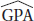= 0.55 + 0.00161 (SAT total) with r = 0.632

What percentage of the variation in GPAs can be explained by looking at the linear relationship between GPAs and SAT scores?

3. In a study of whether the structure of the adult human brain changes when a new skill is learned, the gray matter volume of four individuals was measured before and after learning a new cognitive skill. The resulting scatterplot was: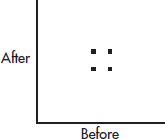The correlation above is 0. Three researchers each run the experiment on a new subject and each obtain an additional data point: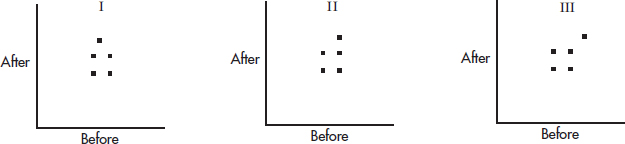Match the above scatterplots with their new correlations.

4. In a study of winning percentage in home games versus average home attendance for professional baseball teams, the resulting regression line is: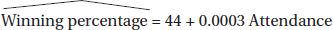What is the residual if a team has a winning percentage of 55% with an average attendance of 34,000?

5. Consider the following scatterplot of midterm and final exam scores for a class of 15 students.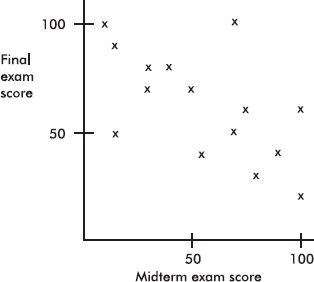Which of the following is incorrect?

6. If every woman married a man who was exactly 2 inches taller than she, what would the correlation between the heights of married men and women be?

7. Suppose the correlation between two variables is r = 0.23. What will the new correlation be if 0.14 is added to all values of the x-variable, every value of the y-variable is doubled, and the two variables are interchanged?

8. Suppose the correlation between two variables is -0.57. If each of the y-scores is multiplied by -1, which of the following is true about the new scatterplot?

9. Consider the set of points {(2, 5), (3, 7), (4, 9), (5, 12), (10, n)}. What should n be so that the correlation between the x- and y-values is 1?

10. Which of the following scatterplots is from data with a correlation coefficient closest to 0?

11. Consider the three points (2, 11), (3, 17), and (4, 29). Given any straight line, we can calculate the sum of the squares of the three vertical distances from these points to the line. What is the smallest possible value this sum can be?

12. Suppose that the scatterplot of log X and log Y shows a strong positive correlation close to 1. Which of the following is true?

13. Consider n pairs of numbers. Suppose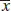= 2, sx = 3,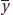= 4, and sy = 5. Of the following, which could be the least squares line?